Latest Banking jobs   »   Reasoning Ability Quiz For IBPS RRB...

# Reasoning Ability Quiz For IBPS RRB PO Prelims 2022- 5th June

Directions (1-5): Study the following information to answer the given question.
In a certain code language,
‘Finance amazon money market’ is written as ‘su da nc ki’,
‘Invest market booster secure’ is written as ‘ph ra tk da’,

Q1. What is the code for ‘amazon’?
(a) da
(b) su
(c) nc
(d) ki
(e) Cannot be determined

Q2. Which of the following is the code for ‘invest business money’?
(a) zo ra ki
(b) ra su mo
(c) tk su ra
(d) ki ra gi
(e) Cannot be determine

Q3. What does ‘ph’ stand for?
(a) finance
(c) automobile
(d) booster
(e) Cannot be determined

Q4. Which of the following is represented by the code ‘da ph nc’?
(a) Booster secure finance
(c) finance market booster
(d) booster invest secure
(e) None of these

Q5. Which of the following may be the possible code for ‘team amazon premium market’?
(a) su ye ph da
(b) mo gi da su
(c) da su mo ph
(d) tk ye su da
(e) None of these

Directions (6-7): Study the information carefully and answer the questions given below.
Rakhi started walking 12km in North direction then takes three consecutive right turns and walked distances 5km, 8km, 4km respectively and reached at point P. From point P, she starts walking in south direction and walks 6km to reach at point G.

Q6. What is the shortest distance between Initial point and G?
(a) 5km
(b) 4km
(c) √5km
(d) 2.5km
(e) None of these

Q7. If point R is 2km west of point P then in which direction of point R with respect to initial point?
(a) North
(b) North-west
(c) South
(d) South-west
(e) None of these

Directions (8-10): Each of these questions is based on the following information:
(i) A # M means A is 3m east of M.
(ii) A & M means A is 7m west of M.
(iii) A % M means A is 4m south of M.
(iv) A * M means A is 6m north of M.

Q8. If the expression ‘S * W & C % B & N % P’ is true, what is the direction of W with respect to N?
(a) North-east
(b) South-East
(c) South-west
(d) North-west
(e) None of these

Q9. If the expression ‘G & H # T * E & R % S’ is true, what is the direction of T with respect to S?
(a) West
(b) North-east
(c) East
(d) North west
(e) None of these

Q10. If the expression ‘A * S & K # L & W % M’ is true, what is the shortest distance between M and S?
(a) 13m
(b) 12m
(c) 10m
(d) 14m
(e) None of these

Directions (11-15): In these questions, a relationship between different elements is shown in the statements. The statements are followed by two conclusions. Give answer

Q11. Statement: A>B; G<D≤E; G≥F>B
Conclusion:
I. G≥A
II. B<E
(a) if only conclusion II is true.
(b) if only conclusion I is true.
(c) if neither conclusion I nor II is true.
(d) if either conclusion I or II is true.
(e) if both conclusions I and II are true.

Q12. Statement: A>T≥J; A≤S=H; I>T
Conclusion:
I. H>J
II. I>S
(a) if both conclusion I and II are true.
(b) if only conclusion I is true.
(c) if neither conclusion I nor II is true.
(d) if either conclusion I or II is true.
(e) if only conclusion II is true.

Q13. Statement: J≥K>L<M≥N; K≥O=T
Conclusion:
I. J>O
II. J=T
(a) if only conclusion II is true.
(b) if either conclusion I or II is true.
(c) if neither conclusion I nor II is true.
(d) if only conclusion I is true.
(e) if both conclusions I and II are true.

Q14. Statement: A> T=N; A>S>R; A<M
Conclusion:
I. R<T
II. N≤R
(a) if only conclusion II is true.
(b) if only conclusion I is true.
(c) if neither conclusion I nor II is true.
(d) if either conclusion I or II is true.
(e) if both conclusions I and II are true.

Q15. Statement: A<B≤C; F<M≤C; C>Q
Conclusion:
I. Q≤F
II. F>Q
(a) if only conclusion II is true.
(b) if either conclusion I or II is true.
(c) if neither conclusion I nor II is true.
(d) if only conclusion I is true.
(e) if both conclusions I and II are true.

Solutions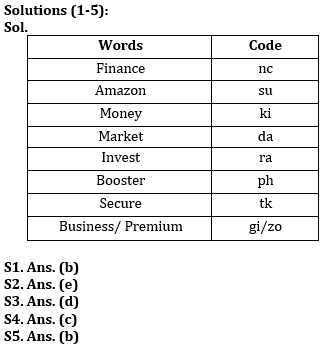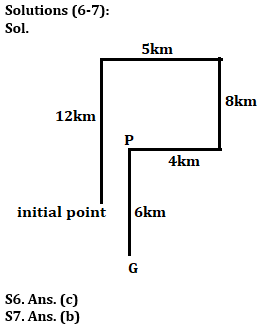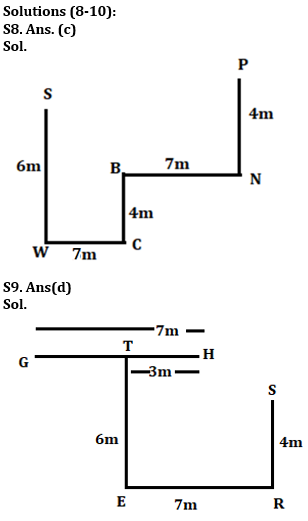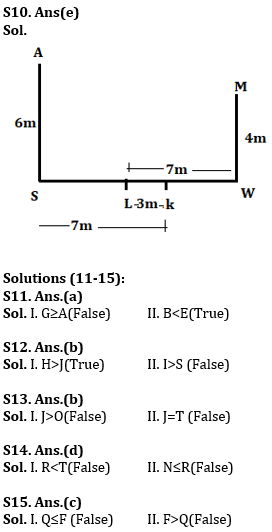#### Congratulations!Incorrect details? Fill the form again here

•Reasoning Ability Quiz For IBPS RRB PO P...
•Important Current Affairs Quiz for Bank ...
•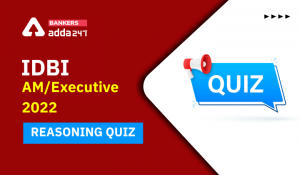Reasoning Ability Quiz For IDBI AM/Execu...
•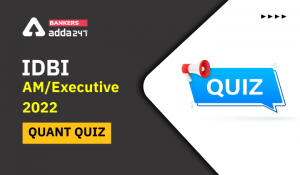Quantitative Aptitude Quiz For IDBI AM/E...
•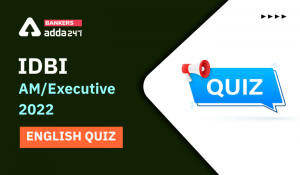English Quizzes For IDBI AM/Executive 20...
•Reasoning Ability Quiz For IDBI AM/Execu...